New Features in Maple 2018 - Dates, Times, and the Calendar Package - Maplesoft# Performance

Performance improvements in Maple 2018 include both broad-based and targeted enhancements.

### Gröbner Bases

Gröbner bases lie at the heart of many fundamental operations, including solving systems of equations and integration, so performance improvements in Gröbner bases results in faster calculations in other areas as well. Maple 2018 includes new optimizations to the F4 algorithm to increase parallel speedup when computing large total degree Gröbner bases.  On a quad core Core i7 with hyperthreading, the new implementation runs 33 percent faster and parallel speedup increases from 1.94x to 2.84x.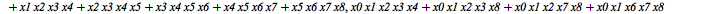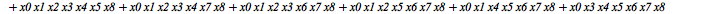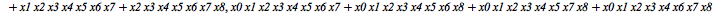memory used=24.08MiB, alloc change=18.98MiB, cpu time=2.73m, real time=25.73s, gc time=0ns

Maple 2018 also includes a new implementation of the FGLM algorithm for converting total degree Gröbner bases to lexicographical order, with improved performance and lower memory requirements.  On this example, the FGLM algorithm runs about 3.5x faster.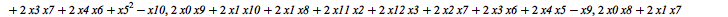memory used=186.80MiB, alloc change=256.00MiB, cpu time=82.03s, real time=26.78s, gc time=93.75ms

### Kernel Improvements

Fast code has been added to the subs command for the case of replacing variables in polynomials with products.  The following types of substitutions run significantly faster on large polynomials.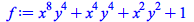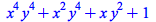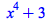Kernel code has been added to speed up tests for polynomials and rational functions using Maple library types.  The examples below run about 6x faster.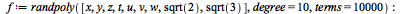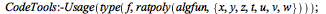memory used=1.98MiB, alloc change=0 bytes, cpu time=15.00ms, real time=13.00ms, gc time=0nsmemory used=1.97MiB, alloc change=0 bytes, cpu time=16.00ms, real time=13.00ms, gc time=0ns### Logic

The SAT solver used by the Satisfy and Satisfiable commands is now MapleSAT, a SAT solver based on MiniSat but with improvements to the variable branching heuristic.  The following example runs about 4x faster in Maple 2018 than Maple 2017.  It encodes an instance of the pigeonhole principle, the fact thatpigeons cannot fit in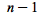holes if each hole can contain at most one pigeon.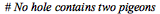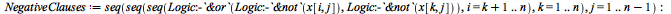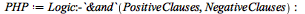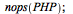memory used=2.02MiB, alloc change=0 bytes, cpu time=18.44s, real time=18.45s, gc time=0ns### Sparse Linear Equations mod p

The msolve command has been updated with a new solver for sparse linear equations for primes up to.  It pivots for structure and sparsity and quickly detects redundant equations in overdetermined systems.  The example below runs over 100x faster.memory used=1.03MiB, alloc change=0 bytes, cpu time=125.00ms, real time=19.00ms, gc time=0ns

### Matrix Inverse with Trig Functions

The inverse of a matrix with trigonometric functions is faster.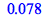### timelimit

The timelimit command is useful for putting a time bound on a computation, preventing it from running longer than you expect. The timelimit command now has virtually no overhead.

 > f := proc(x)    description "Number of Primes less than x";    global i,j;    i:=0:    for j to x do        if isprime(j) then            i := i + 1;        end if;    end do:    return i; end proc:
 > timelimit(0.5,f(1000000));
 > printf("The number of primes less than %a is %a. This was computed in %.2f s.", j, i, 0.5);
 The number of primes less than 150743 is 13909. This was computed in 0.50 s.